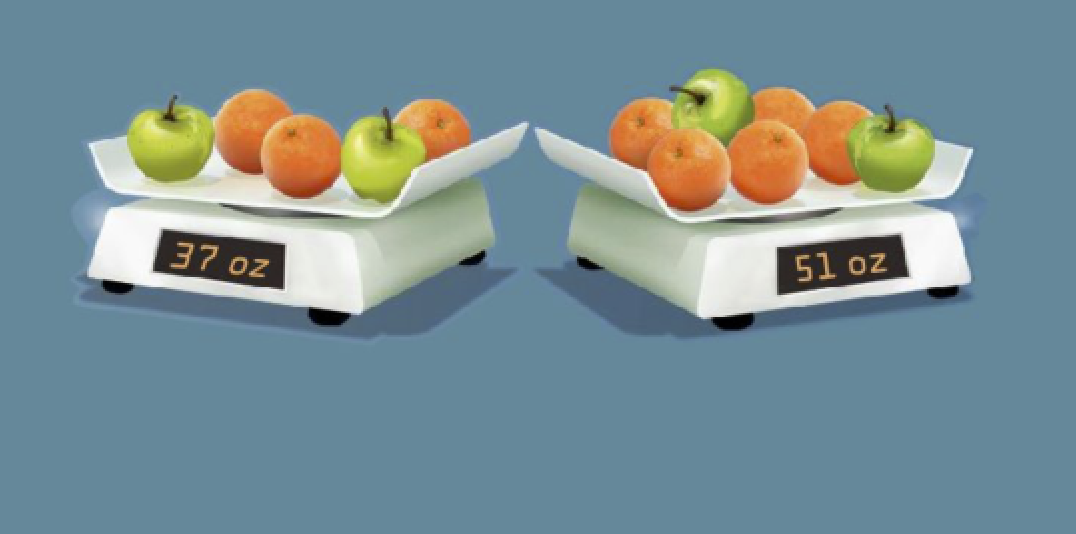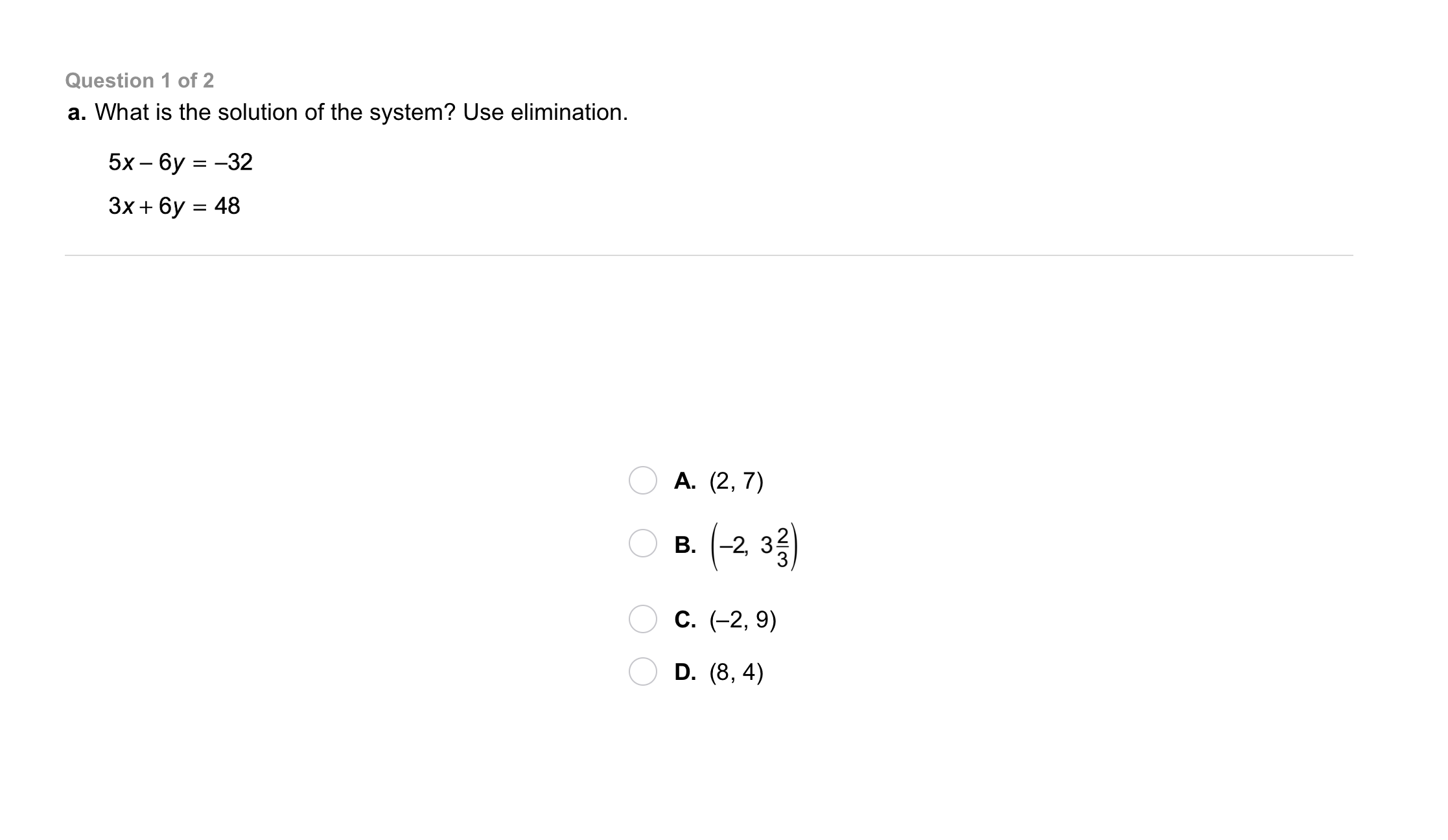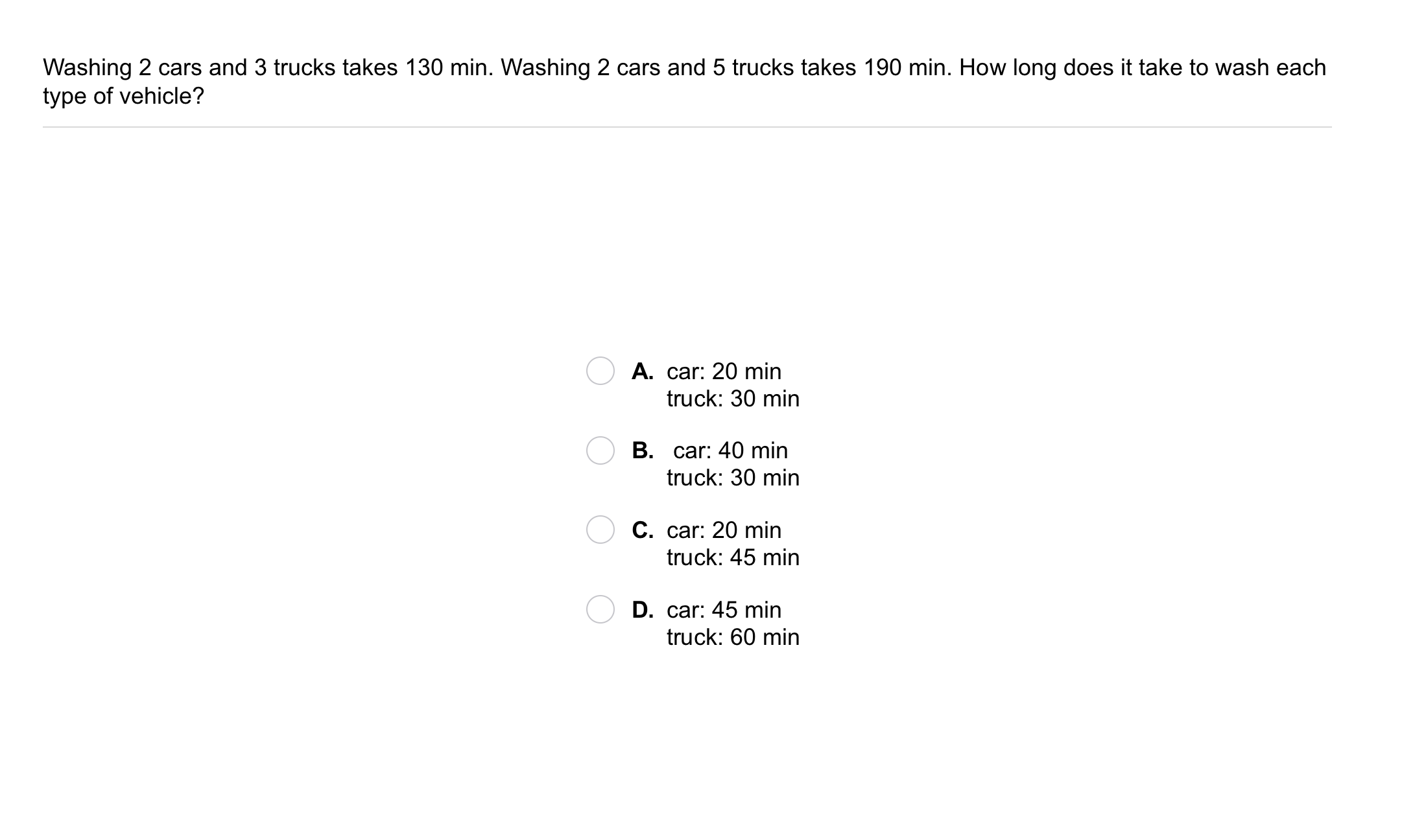Algebra 1 6-3 Guided Practice: Solving Systems Using Elimination
starstarstarstarstarstarstarstarstarstar
by Matthew Richardson
| 19 Questions1
2
1
10
Solve It! A cafeteria sells fresh fruit by weight. All apples weigh the same, and all oranges weigh the same. What is the weight of an apple? Enter your response using the same format shown on the scales, with a space between the measurement and oz.
2
10
Solve It! In this same scenario, what is the weight of an orange? Enter your response using the same format shown on the scales, with a space between the measurement and oz.3
4
5
3
10
Take Note: Review: Describe the process of solving systems of equations by graphing.
4
10
Take Note: Review: Describe the process of solving systems of equations using the substitution method.
5
10
Take Note: Describe the process of solving systems of equations using the elimination method.6
6
10
Problem 1 Got It?
A
B
C
D7
7
10
Problem 1 Got It?
A
B
C
D8
9
8
10
Take Note: Consider the equation below.
Write an equation that can be added to the given equation to cancel the x-terms completely.
9
10
Take Note: Consider the equation below.
Write an equation that can be subtracted from the given equation to cancel the y-terms completely.10
10
10
Problem 2 Got It?
A
B
C
D11
11
10
Take Note: Consider the system of linear equations below:
2x+3y=11\\x-2y=-5
The equations do not yet have equal or opposite coefficients of either x or y.
Describe the first step that is necessary if solving this system with the elimination method. Hint: It will create equal or opposite numbers of x's or y's.
13
10
Problem 3 Got It? Revise and solve the system using elimination. Show your work on the canvas.
In this case, revise means to multiply one or both equations by a number to establish an equivalent system for solving by elimination.
14
5
Problem 3 Got It? Is the solution of the revised system also the solution of the original system? If not, consider checking your work.
Yes, the solution applies to both systems.
No, the solution only applies to the revised system.
15
10
Problem 4 Got It? How can you use the Multiplication Property of Equality to change an equation in the system below in order to solve it using elimination?

16
10
Problem 4 Got It? Revise and solve the system using elimination. Show your work on the canvas.

17
5
Problem 4 Got It? Is the solution of the revised system also the solution of the original system? If not, consider checking your work.
Yes, the solution applies to both systems.
No, the solution only applies to the revised system.18
18
10
Problem 5 Got It?
A
B
C19
19
10
Take Note: Summarize the content of this lesson.